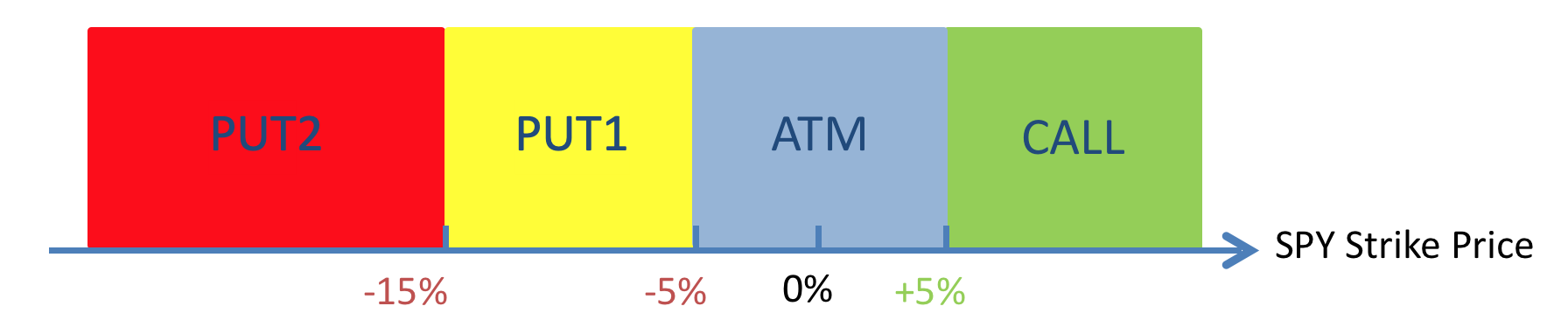## SPIKES Settlement Analytics - A Primer

March 8, 2019

This primer provides a detailed explanation of the analytics conducted on this site, as well as outlining key concepts and terminology. A pdf of the primer can be downloaded here.

#### Settlement Reference Price (SRP) and Cash Reference Price (CRP)

The SRP is the price of each SPY option that is used in the SPIKES settlement calculation. The CRP on the other hand is the SPY option price used in the SPIKES cash index calculation. By comparing the two we may be able to see where possible discrepancies lie.

After each SPIKES settlement the exchange publishes alongside the SRPs two sets of CRP values, namely CRP1 and CRP2. CRP1 is the CRP when the value of each SRP is determined. The timestamp is unique for each SPY option. CRP2 is the CRP at the SPIKES settlement time, when the SPKCS value is calculated. This timestamp is the same for all SPY options and it occurs after all CRP1 timestamps. The relationship between the SRPs and the CRPs are illustrated in the following diagram:

If all goes well the settlement process should take a few seconds to complete from start to finish. The SRP values should be very close to the CRPs for each SPY option, and so should the SPKCS value be very close to the hypothetical settlement values calculated using the CRP1 and CRP2 inputs. These are published by the exchange and are shown in the first table on our analytics webpage.

Furthermore we may calculate potentially interesting statistics such as the CRP range and the SRP position, as illustrated below.

#### SPY Options Volume at the Settlement Auction

Note: the analysis in this section applies after SPIKES futures are listed and become sufficiently liquid.
Market makers hold inventories of SPIKES futures during their regular trading operations and need to hedge their exposures on settlement date. Since the SPIKES index is constructed using the variance swap methodology, there exists a static portfolio of SPY options that will replicate the SPIKES future more or less exactly at settlement time.

Typically market makers will put a strategy order, comprising of SPY call and put options of varying quantities and covering a wide range of at-the-money (ATM) and out-of-the-money (OTM) strike prices, into the SPIKES settlement auction to offset their risk in SPIKES futures. If they are long SPIKES futures, they will be selling SPY options at the auction. Conversely if they are short SPIKES futures, they will be buying SPY options.

The number of SPY options required at each strike price is given explicitly by the SPIKES index formula which is derived using the variance swap approach:

For our purposes we only need to consider the summation term (highlighted in bold). Here Ki and pi are the strike and option prices respectively; ∆Ki is the gap between adjacent strikes. Hence for each SPY option the portfolio weight is proportional to ∆Ki and inversely proportional to Ki ^2.

In order to compute the volume projection for each strike, we first estimate traded volume for the ATM strike and then apply the following formula to obtain estimates for the other strikes:

If these strategy orders are dominant at the SPIKES settlement auction, we expect to see SPY options traded volume conforming closely to the outlined theoretical projections, as shown in the example below. Notice the OTM puts have significantly higher volume than the higher strikes.

To quantify how different actual traded volume is compared to projections on a macroscopic level, we calculate the volume projection ratio as the ratio between total SPY options traded in the SPIKES settlement auction and the total projected volume. Further investigation is warranted if the result is significantly different from unity.

#### Volume and Price Analysis by SPY Strike Range

Since different SPY strikes carry vastly different weights in the SPIKES settlement value (largely due to the inverse squared factor), we need to zoom in to analyse both volume and price across the SPY options strike range. We partition this into four distinct sections as shown below.Figure 4. SPIKES settlement SPY option strike ranges for analysis purposes

For each SPY strike range we summarise the number of strikes, auction volume per strike, volume relative to last trading day’s total volume, volume relative to open interest, projection ratio, as well as median CRP range and SRP position.

Another interesting exercise is to see how different the SPIKES settlement value would be if some of the SRPs are replaced by the CRPs. We will do so for each strike range, one at a time, as outlined above. We can study the results and find where possible distortions may come from, especially when the SPKCS value is outside the range of possible SPIKES values when the CRPs are used in one of the strike ranges.

The following box-plot example illustrates how this “sensitivity” analysis works. Each box is bounded by alternative SPIKES settlement values using only the CRP1 or CRP2 inputs for each SPY option. The vertical lines above and below each box indicate the upper and lower bounds if for each SPY option, either the maximum or minimum CRP value is used in the SPIKES settlement calculation.